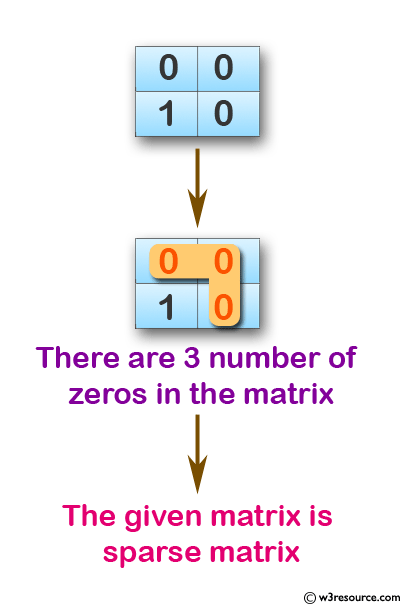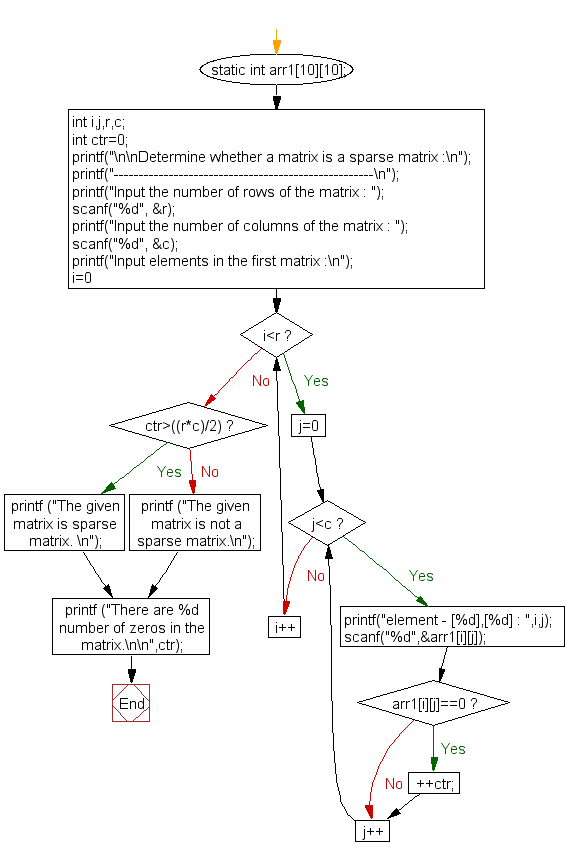﻿ C exercises: Determine whether a matrix is a sparse matrix - w3resource# C Exercises: Determine whether a matrix is a sparse matrix

## C Array: Exercise-29 with Solution

Write a program in C to accept a matrix and determine whether it is a sparse matrix.

Pictorial Presentation:Sample Solution:

C Code:

``````#include <stdio.h>

/*A sparse martix is matrix which  has more zero elements than nonzero elements */
void main ()
{
static int arr1;
int i,j,r,c;
int ctr=0;
printf("\n\nDetermine whether a matrix is a sparse matrix :\n");
printf("----------------------------------------------------\n");
printf("Input the number of rows of the matrix : ");
scanf("%d", &r);
printf("Input the number of columns of the matrix : ");
scanf("%d", &c);
printf("Input elements in the first matrix :\n");
for(i=0;i<r;i++)
{
for(j=0;j<c;j++)
{
printf("element - [%d],[%d] : ",i,j);
scanf("%d",&arr1[i][j]);
if (arr1[i][j]==0)
{
++ctr;
}
}
}
if (ctr>((r*c)/2))
{
printf ("The given matrix is sparse matrix. \n");
}
else
printf ("The given matrix is not a sparse matrix.\n");

printf ("There are %d number of zeros in the matrix.\n\n",ctr);
}
```
```

Sample Output:

```Determine whether a matrix is a sparse matrix :
----------------------------------------------------
Input the number of rows of the matrix : 2
Input the number of columns of the matrix : 2
Input elements in the first matrix :
element - , : 0
element - , : 0
element - , : 1
element - , : 0
The given matrix is sparse matrix.
There are 3 number of zeros in the matrix.
```

Flowchart:C Programming Code Editor:

Improve this sample solution and post your code through Disqus.

What is the difficulty level of this exercise?

﻿